# Indian Talent Olympiad - IMO PDF Sample Papers for Class 1

Class 1 sample paper & practice questions for International Maths Olympiad (IMO) level 1 are given below. Syllabus for level 1 is also mentioned for these exams. You can refer these sample paper & quiz for preparing for the exam.#### Resources:

##### Sample Questions from Olympiad Success:
 Q.1 Q.2 Q.3 Q.4 Q.5 Q.6 Q.7 Q.8 Q.9 Q.10
 Q.1 Write 76 in words. a) Seventy six b) Fifty five c) Seventy five d) Forty five
 Q.2 How many pencils are there in the given figure?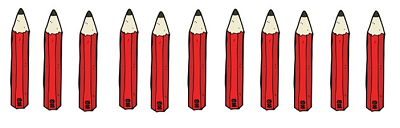a) 12 b) 7 c) 11 d) 16
 Q.3 What is the total value of the coins?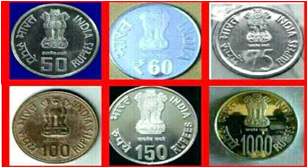a) 1455 Rs b) 1500 Rs c) 1435 Rs d) 1400 Rs
 Q.4 What is the sum of all sides given in the figure?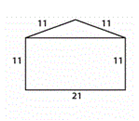a) 44 b) 55 c) 65 d) 75
 Q.5 Write 64 in words. a) Sixty four b) Fifty five c) Seventy five d) Forty five
 Q.6 What shapes are used to make rocket?a) Rectangle, square, triangle, oval. b) Circle, rectangle, square. c) Rectangle, square, triangle. d) Circle, rectangle, square, triangle.
 Q.7 Measure the object using blocks.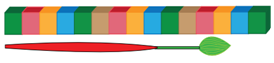a) 12 b) 15 c) 14 d) 13
 Q.8 Subtract the given number: 71 - 25 + 35 = ……….. a) 61 b) 51 c) 71 d) 81
 Q.9 What is the name of given shape?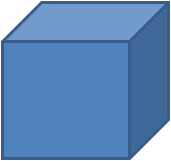a) Square b) Rectangle c) Cuboid d) Cube
 Q.10 What is the numeral of seventy nine? a) 79 b) 51 c) 41 d) 89Sample PDF of Indian Talent Olympiad - International Maths Olympiad (IMO) PDF Sample Papers for Class 1:

 Q.1 )a Q.2 )c Q.3 )c Q.4 )c Q.5 )a Q.6 )d Q.7 )d Q.8 )d Q.9 )d Q.10 )a

Q.1 : a | Q.2 : c | Q.3 : c | Q.4 : c | Q.5 : a | Q.6 : d | Q.7 : d | Q.8 : d | Q.9 : d | Q.10 : a